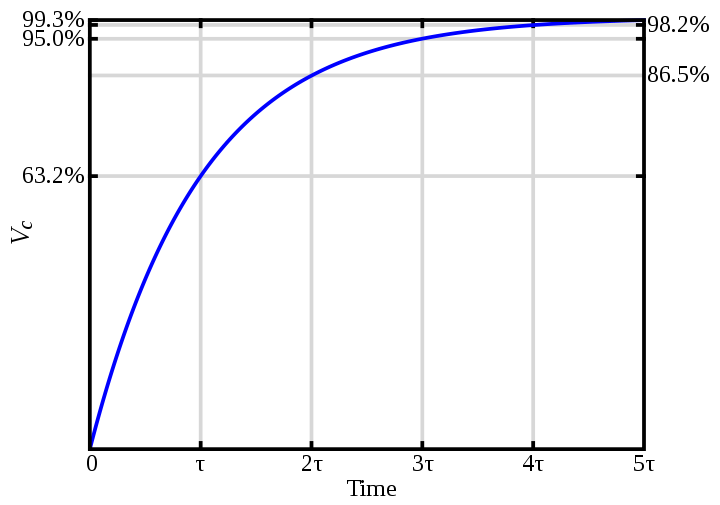A resistor–capacitor circuit (RC circuit), or RC filter or RC network, is an electric circuit composed of resistors and capacitors. It may be driven by a voltage or current source and these will produce different responses. A first order RC circuit is composed of one resistor and one capacitor and is the simplestIn an RC circuit, the product of the resistance in ohms and the capacitance in farads is called the time constant, T = RC. A capacitor will charge or discharge to 63.2 % of its final value in 1 T. It takes about 5 T to charge to the source voltage.

t = RC
example:
R = 5000Ω
C = 10000µF or 0.01F

5000Ω x 10000µF = 5000Ω x 0.01F = 50 seconds to charge to 63.2%.  5T (full charge) = 5 * 50 seconds or 250 seconds.